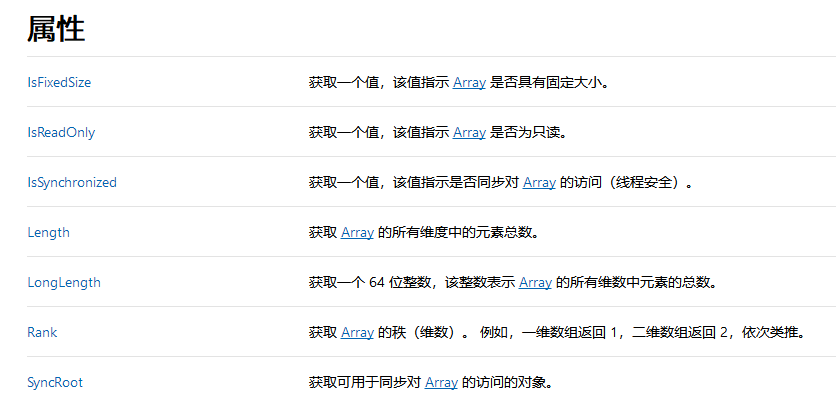# C# 多维数组 交错数组的区别，即 [ , ] 与 [ ][ ]的区别

int [,] test1 = new int [3,3];


或声明时即赋值，由系统推断长度

int [,] test1 = {
{1,2,3},
{1,2,3},
{1,2,3},
};

int [][] test1 = new int[];
int [][] test1 = new int[][];　　　　//注意，此的声明方式是错的

        int [][] test1 = {
new int[] {1,2,3,4},
new int[] {1,2,3},
new int[] {1,2}
}；


多维数组与交错数组 二者的相同、区别

int [,] test1 = {
{1,2,3,4},
{1,2,3},
{1,2}
};         //这样是错的，长度必须一致
int [,] test1 = new int [4,5] {
{1,2,3,4,5},
{1,2,3},
{1,2,3}
}；　　　　　　　　//这样也是错误的，长度必须一致，必须为每一个位置赋值

这一点C#与C语言有所区别，C语言可以不全赋值，没有赋值的位置系统默认为0。

int [,] test1 = {
{1,2,3},
{1,2,3},
{1,2,3}
};


初始化交错数组

  int [][] test1 = {
new int[] {1,2,3,4},　　　　 //new int {1,2,3,4}
new int[] {1,2,3},  　　　　//new int {1,2,3}
new int[] {1,2}
}；


注意，在里面有 new int[]，这正是交错数组的特性。交错数组是由数组构成的数组，交错数组要求为内部的每个数组都创建实例。

即交错数组的每一维都是一个实例，每一个实例为一个数组。

        int [,] test1 = {
{1,2,3},
{1,2,3},
{1,2,3}
};
Console.WriteLine(test1.Length);
输出为   9


       int [][] test1 = {
new int[] {1,2,3},
new int[] {1,2,3},
new int[] {1,2,3},
};
Console.WriteLine(test1.Length);   输出为 3

https://www.jb51.net/Special/265.htmhttps://docs.microsoft.com/zh-cn/dotnet/api/system.array?view=netframework-4.7.2

### 使用数组初始化类型

using System.Collections.Generic;        //头部引入

//main中的代码
static void Main(string[] args)
{
List<string> list = new List<string>();

Console.ReadKey();
}


        static void Main(string[] args)
{
List<string> list = new List<string>();
//增加
list.Add("a");
list.Add("b");
list.Add("c");
list.Add("d");
list.Add("e");
list.Add("f");
list.Add("g");
Console.ReadKey();
}

List<string> list = new List<string>(){"a","b","c","d","e","f"};

List<string> list = new List<string>{"a","b","c","d","e","f"};

//以上两种方法都可以，注意后面有没有 ()

试试交错数组

1，在 program  所在的命名空间中写一个类

    public class Test
{
public int x;
public int y;
public void What()
{
Console.WriteLine(x + y);
}
}

2，在 Main 方法中

       static void Main(string[] args)
{
List<Test> list = new List<Test>()
{
new Test{x=1,y=6},
new Test{x=8,y=6},
new Test{x=4,y=8},
new Test{x=5,y=7},
new Test{x=3,y=3},
new Test{x=6,y=6},
new Test{x=9,y=666},
new Test{x=7,y=6},
};
Console.ReadKey();
}

    public class Test
{
public int x;
public int y;
public void What()
{
Console.WriteLine(x + y);
}
}
class Program
{
static void Main(string[] args)
{
List<Test> list = new List<Test>()
{
new Test{x=1,y=6},
new Test{x=8,y=6},
new Test{x=4,y=8},
new Test{x=5,y=7},
new Test{x=3,y=3},
new Test{x=6,y=6},
new Test{x=9,y=666},
new Test{x=7,y=6},
};
Console.ReadKey();
}
}

posted @ 2018-11-09 17:00 痴者工良 阅读(...) 评论(...) 编辑 收藏